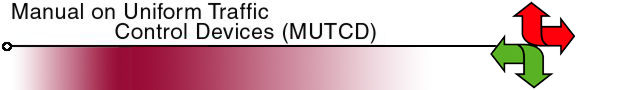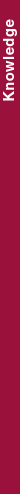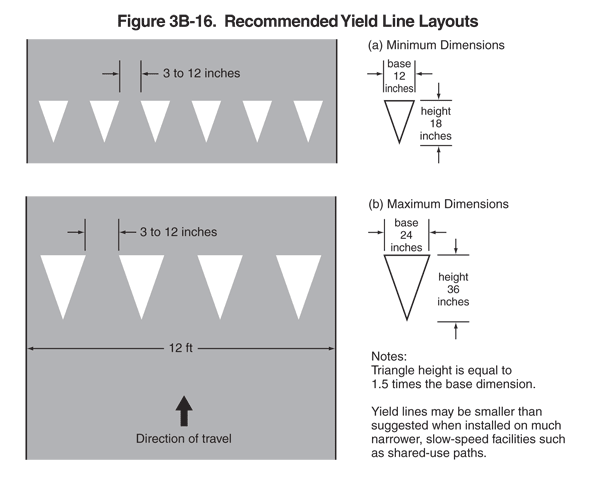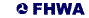Search MUTCD Site:# 2009 Edition Part 3 Figure 3B-16. Recommended Yield Line LayoutsFigure 3B-16. Recommended Yield Line Layouts

This figure illustrates two examples of yield line layouts.

Notes state that "triangle height is equal to 1.5 times the base dimension. Yield lines may be smaller than suggested when installed on much narrower, slow-speed facilities such as shared-use paths."

The figure shows examples (a) and (b):

• Example (a) is labeled "minimum dimensions." The figure shows a row of six solid white isosceles triangles. The base of each is shown as a dimension of 12 inches wide, and the height of each is shown as a dimension of 18 inches long, spaced 3 to 12 inches apart.
• Example (b) is labeled "maximum dimensions." The figure shows a row of four solid white isosceles triangles. The base of each is shown as a dimension of 24 inches wide, and the height of each is shown as a dimension of 36 inches long, spaced 3 to 12 inches apart. The pavement is shown as a dimension of 12 ft wide. A black arrow shows the direction of travel, which is toward the points of the triangles.

Back to Chapter 3BUnited States Department of Transportation - Federal Highway Administration